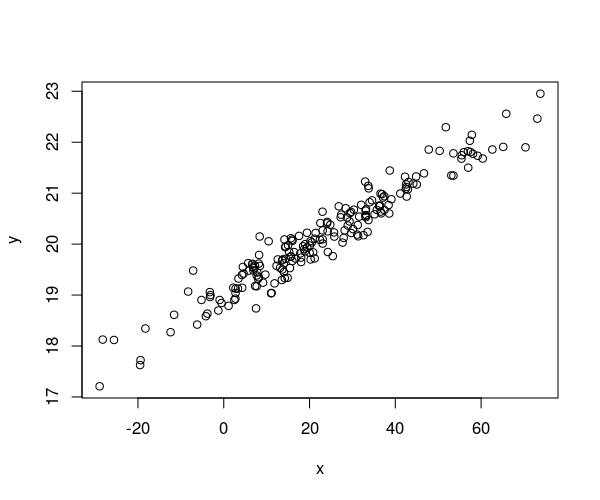## Exam 1

1. #### Question

The following figure shows a scatterplot. Which of the following statements are correct?1. The absolute value of the correlation coefficient is at most $0.8$.
2. The standard deviation of $X$ is at least $6$.
3. For $X=17.1$, $Y$ can be expected to be about 19.9 .
4. The scatterplot is standardized.
5. The mean of $Y$ is at least $30$.

#### Solution

1. False. A strong association between the variables is given in the scatterplot. Hence the absolute value of the correlation coefficient is close to $1$ and therefore larger than $0.8$.
2. True. The standard deviation of $X$ is about equal to $20$ and is therefore larger than $6$.
3. True. The regression line at $X=17.1$ implies a value of about $Y=19.9$.
4. False. The scatterplot is not standardized, because $X$ and $Y$ do not both have mean $0$ and variance $1$.
5. False. The mean of $Y$ is about equal to $20$ and hence is smaller than $30$.